# Unit Rates Vocabulary A rate is a ratio

• Slides: 9Unit RatesVocabulary • A rate is a ratio that compares two quantities measured in different units. • The unit rate is the rate for one unit of a given quantity. Unit rates have a denominator of 1.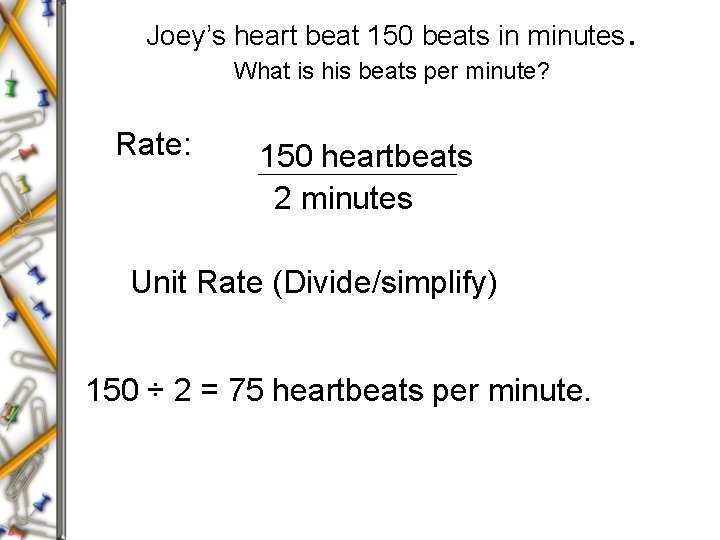Joey’s heart beat 150 beats in minutes. What is his beats per minute? Rate: 150 heartbeats 2 minutes Unit Rate (Divide/simplify) 150 ÷ 2 = 75 heartbeats per minute.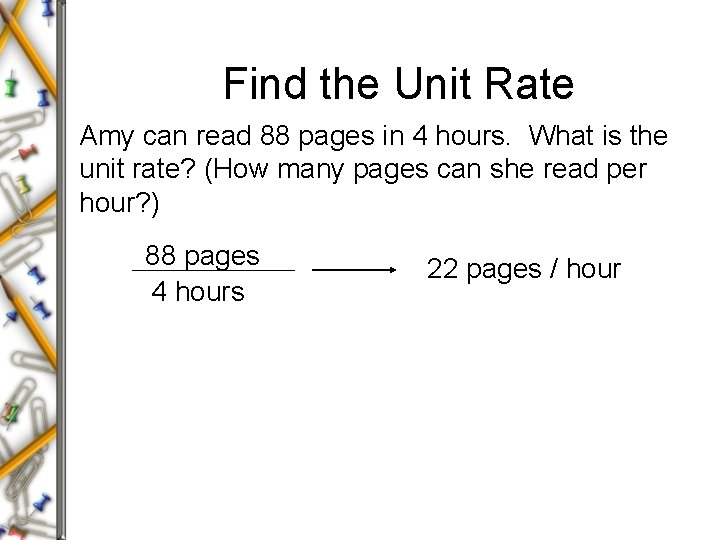Find the Unit Rate Amy can read 88 pages in 4 hours. What is the unit rate? (How many pages can she read per hour? ) 88 pages 4 hours 22 pages / hour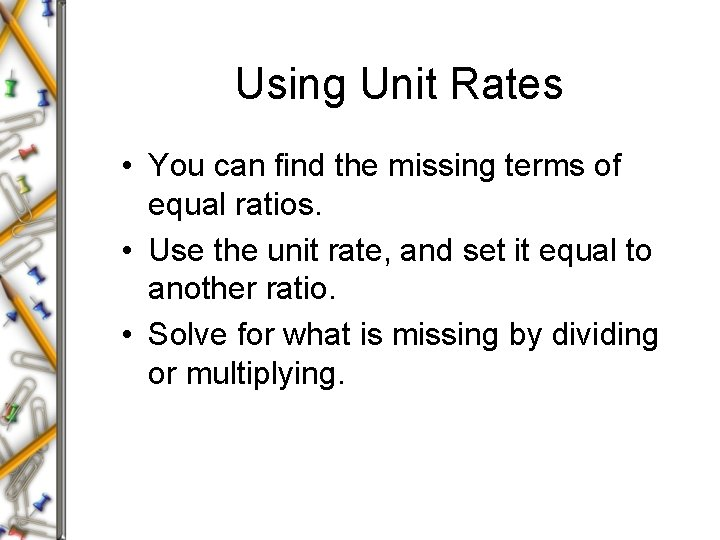Using Unit Rates • You can find the missing terms of equal ratios. • Use the unit rate, and set it equal to another ratio. • Solve for what is missing by dividing or multiplying.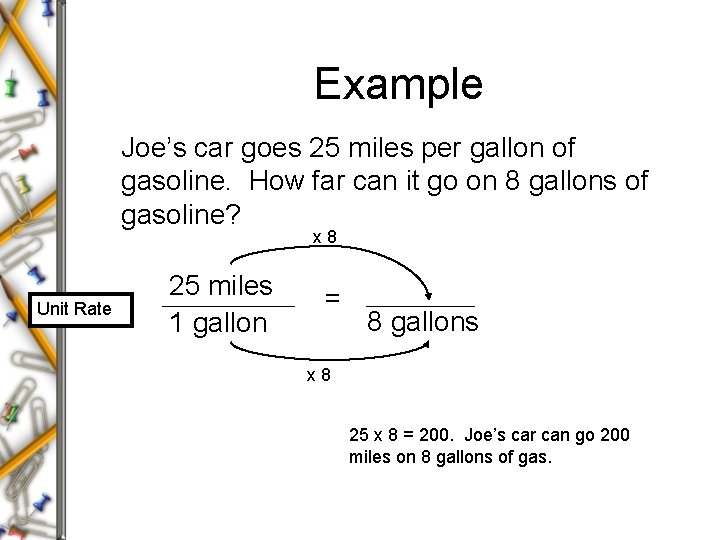Example Joe’s car goes 25 miles per gallon of gasoline. How far can it go on 8 gallons of gasoline? x 8 Unit Rate 25 miles 1 gallon = 8 gallons x 8 25 x 8 = 200. Joe’s car can go 200 miles on 8 gallons of gas.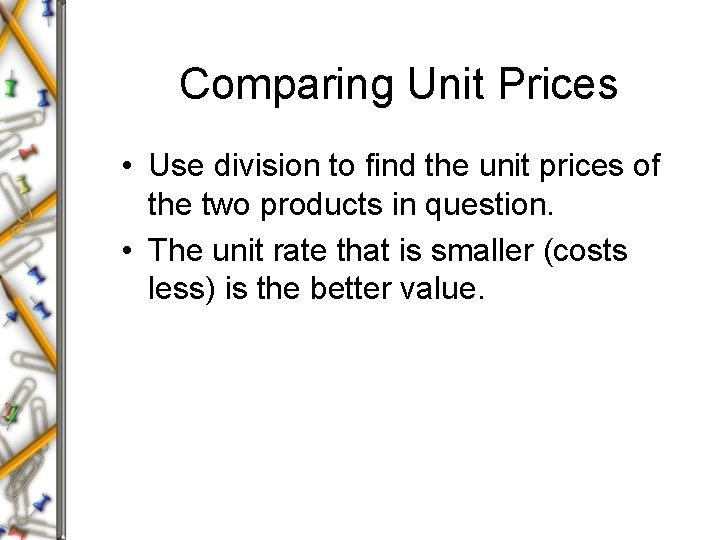Comparing Unit Prices • Use division to find the unit prices of the two products in question. • The unit rate that is smaller (costs less) is the better value.Example Juice is sold in two different sizes. A 48 -fluid ounce bottle costs \$2. 07. A 32 -fluid ounce bottle costs \$1. 64. Which is the better buy? \$2. 07 48 fl. oz. \$1. 64 32 fl. oz. 0. 043125 0. 05125 The 48 fl. oz. bottle is the better value. \$0. 04 per fl. oz. \$0. 05 per fl. oz.Homework Time